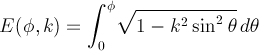Computes the incomplete elliptic integral of the second kind.

Namespace:  Meta.Numerics.Functions
Assembly:  Meta.Numerics (in Meta.Numerics.dll) Version: 4.1.4Syntax
```public static double EllipticE(
double phi,
double k
)```

#### Parameters

phi
Type: SystemDouble
k
Type: SystemDouble
The elliptic modulus, which must lie between zero and one.

#### Return Value

Type: Double
The value of E(phi,k).Remarks

The incomplete elliptic integral of the second kind is:It appears in the Legendre reduction of integrals of rational funtions.

Be aware that some authors use the the parameter m = k2 instead of the modulus k.See Also